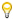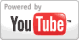Graphing Systems of Equations - Algebra Lesson
Grade: 7 - 12 | In this lesson, students learn to solve a system of linear equations by graphing. The first step is to graph each of the given equations, then find the point of intersection of the two lines, which is the solution to the system of equations.Add to My DashboardAlgebra
For Other Topics :Quiz Games by TopicInteractive DiagramsFlow Chart GamesVocabulary GamesSlide PuzzlesJigsaw PuzzlesCreate PresentationsBrain GamesVideos by Topic### Search Videos & Games